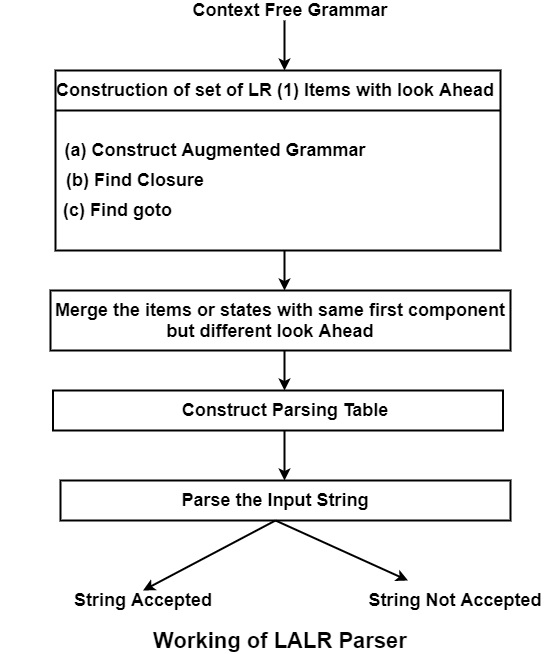# What is LALR (1) Parser?

LALR Parser is Look Ahead LR Parser. It is intermediate in power between SLR and CLR parser. It is the compaction of CLR Parser, and hence tables obtained in this will be smaller than CLR Parsing Table.

Here, first of all, we will construct LR (1) items. Next, we will look for the items having the same first component, and they are merged to form a single set of items. It means the states have the same first component, but the different second component can be integrated into a single state or item.

For Example.

Suppose if

I4: C → d ∙ , c | d

I7: C → d ∙ , $Both items or states (I4 and I7) have the same first component, i.e., d ∙ , but a different second component, i.e., c | d in I4 and$ in I7.

∴ these states can be merged to give

I47: C → d ∙ , c |d | \$

Construction of LALR Parsing Table

Algorithm

Input − Augmented Grammar G′

Output − LALR Parsing Table

Method

• Construct LR (1) set of items, i.e., construct

C = {I0, I1, I2 … . . In}

• Select the similar states having the same core, or first component and merge them into one.

Let C′ = {J0, J1, J2 … . . Jm} be the resulting set.

• Construct Parsing Action for state J1 similar to CLR construction. If there is a conflict in the Parsing Table, the algorithm can be considered to fail to produce an LALR parser.

• Construct goto actions as below.

Let goto [J,∗] = K where J is the union of one or more states of C.

i.e., J = I1 ∪ I2 … .∪ Im, then

then K = goto (I1,∗) ∪ goto (I2,∗) … .∪ goto (Im,∗)

Working of LALR ParserLALR Grammar − A grammar where the LALR parsing table has no multiply represented entries are said to be LALR (1) or LALR grammar.